# Thank You×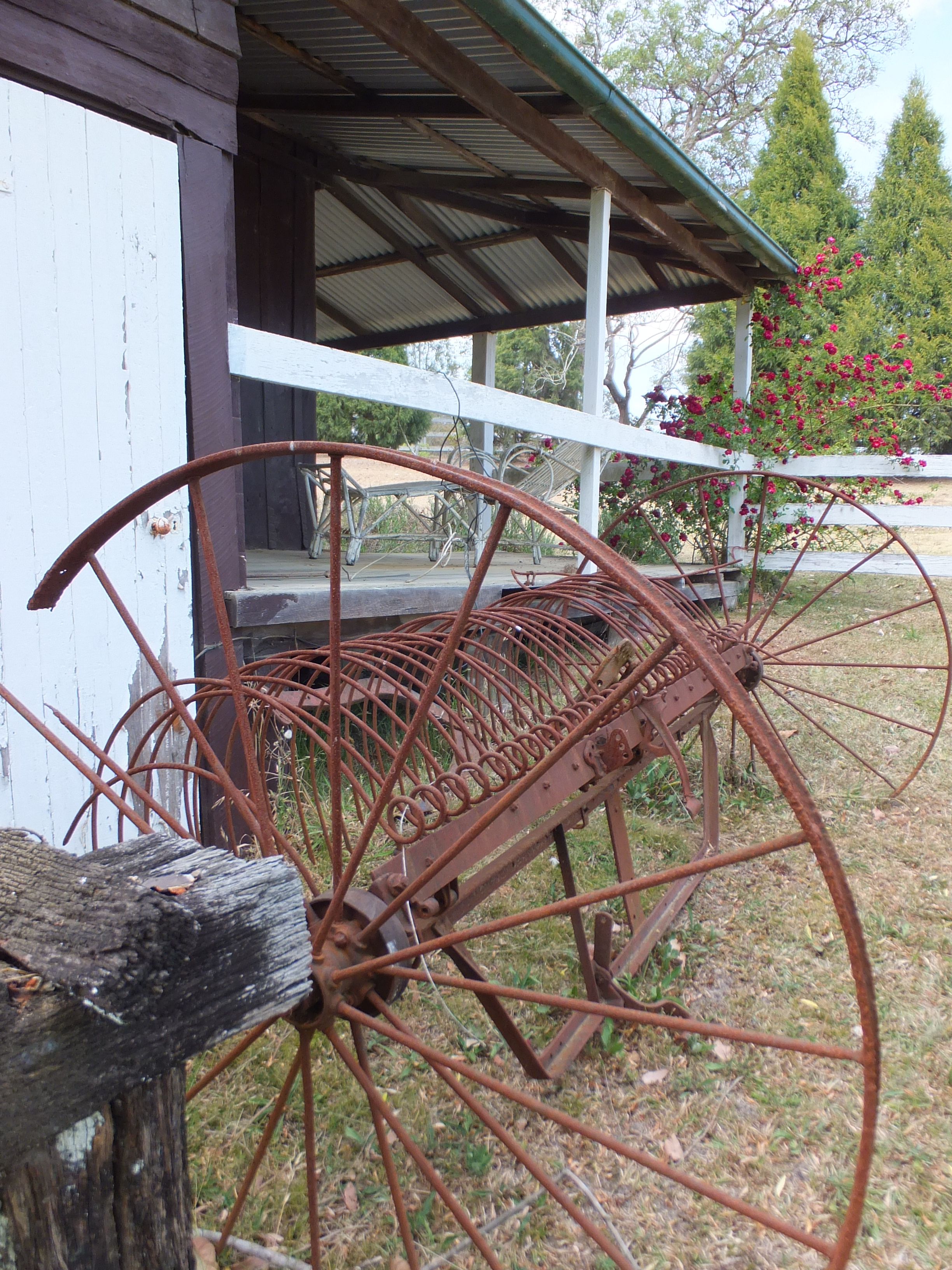×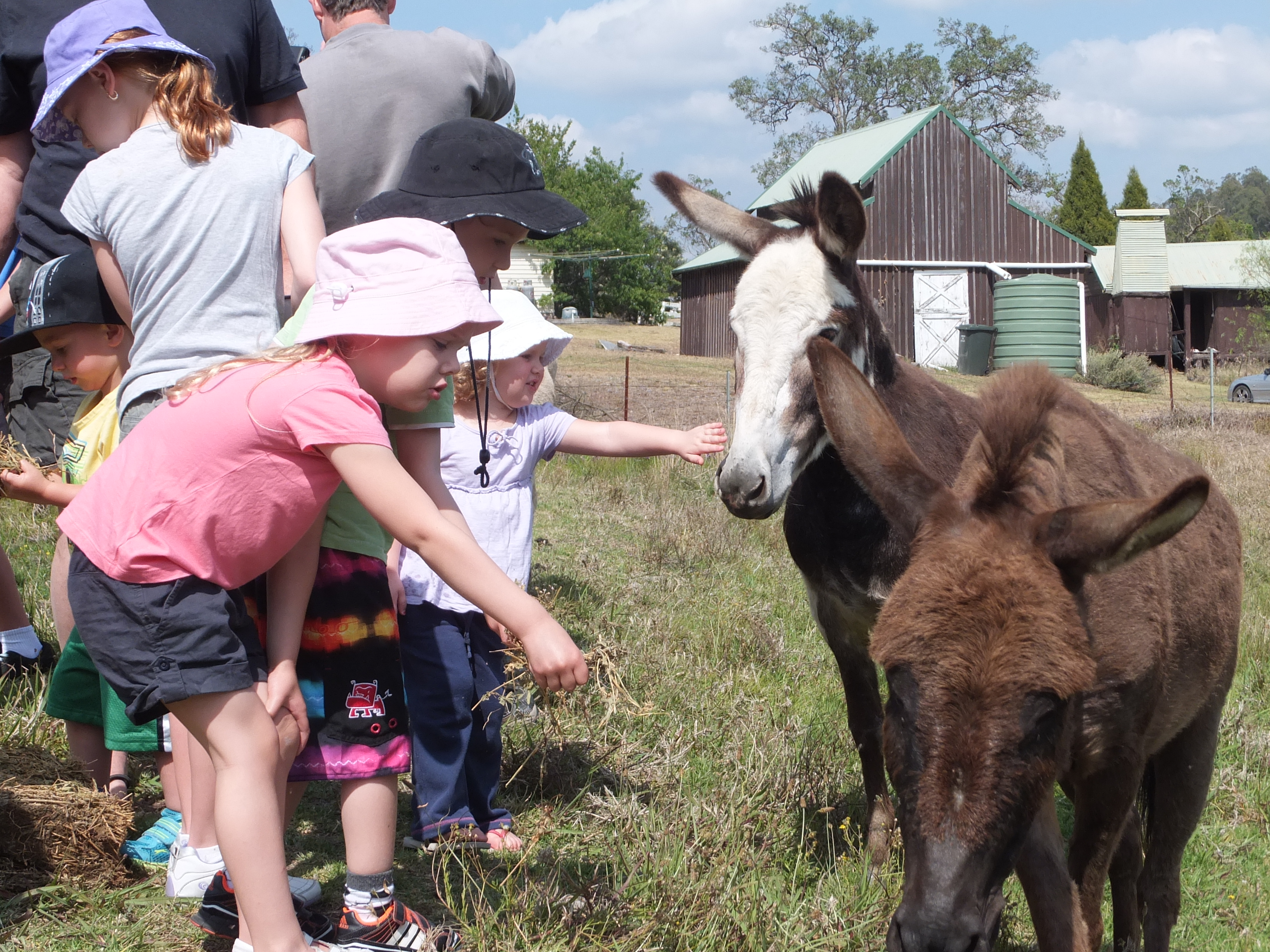×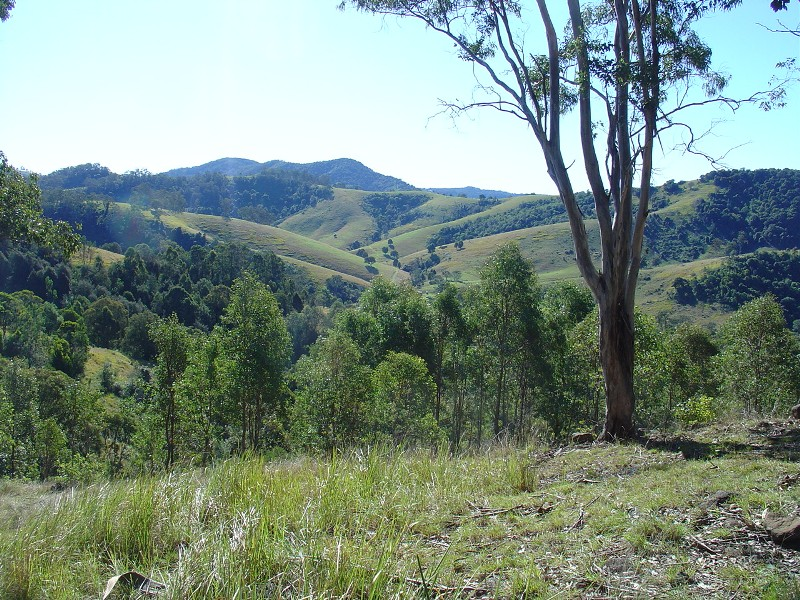×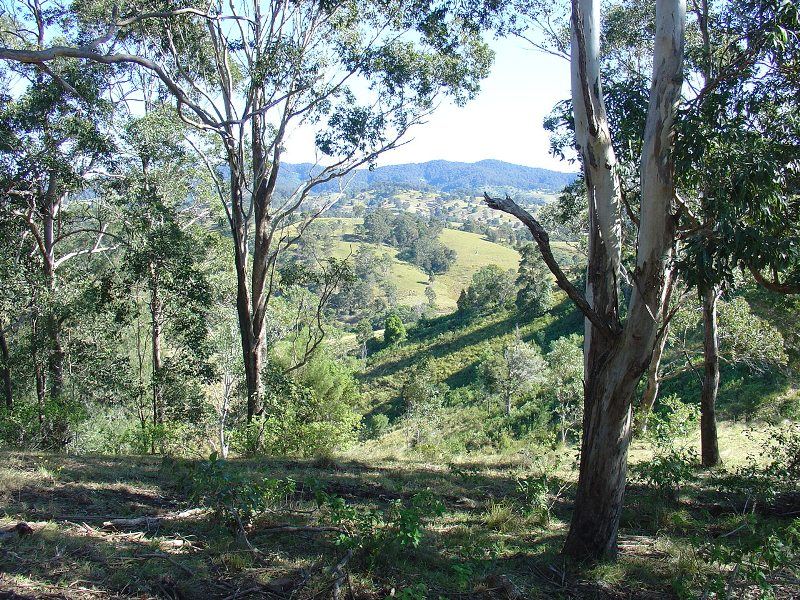××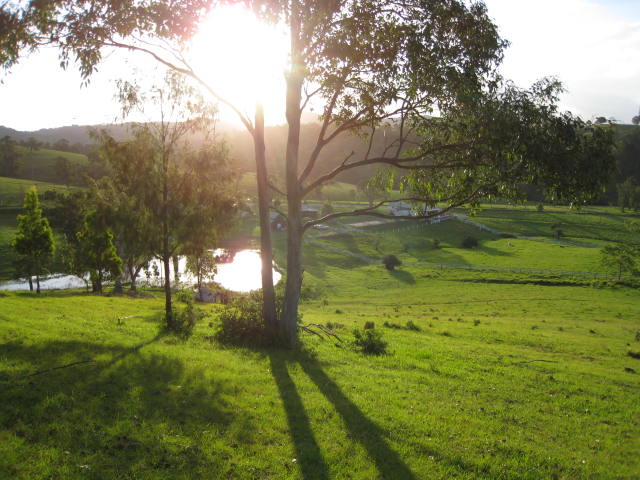×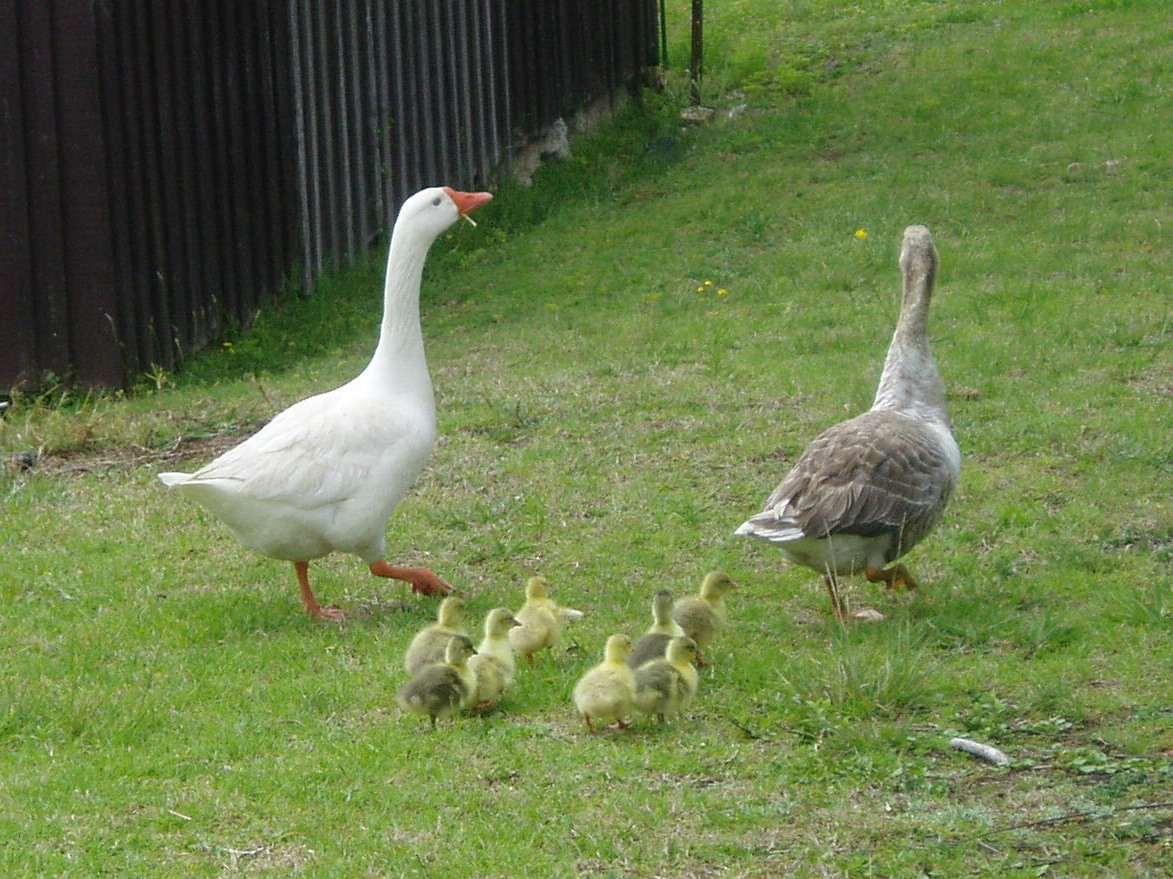××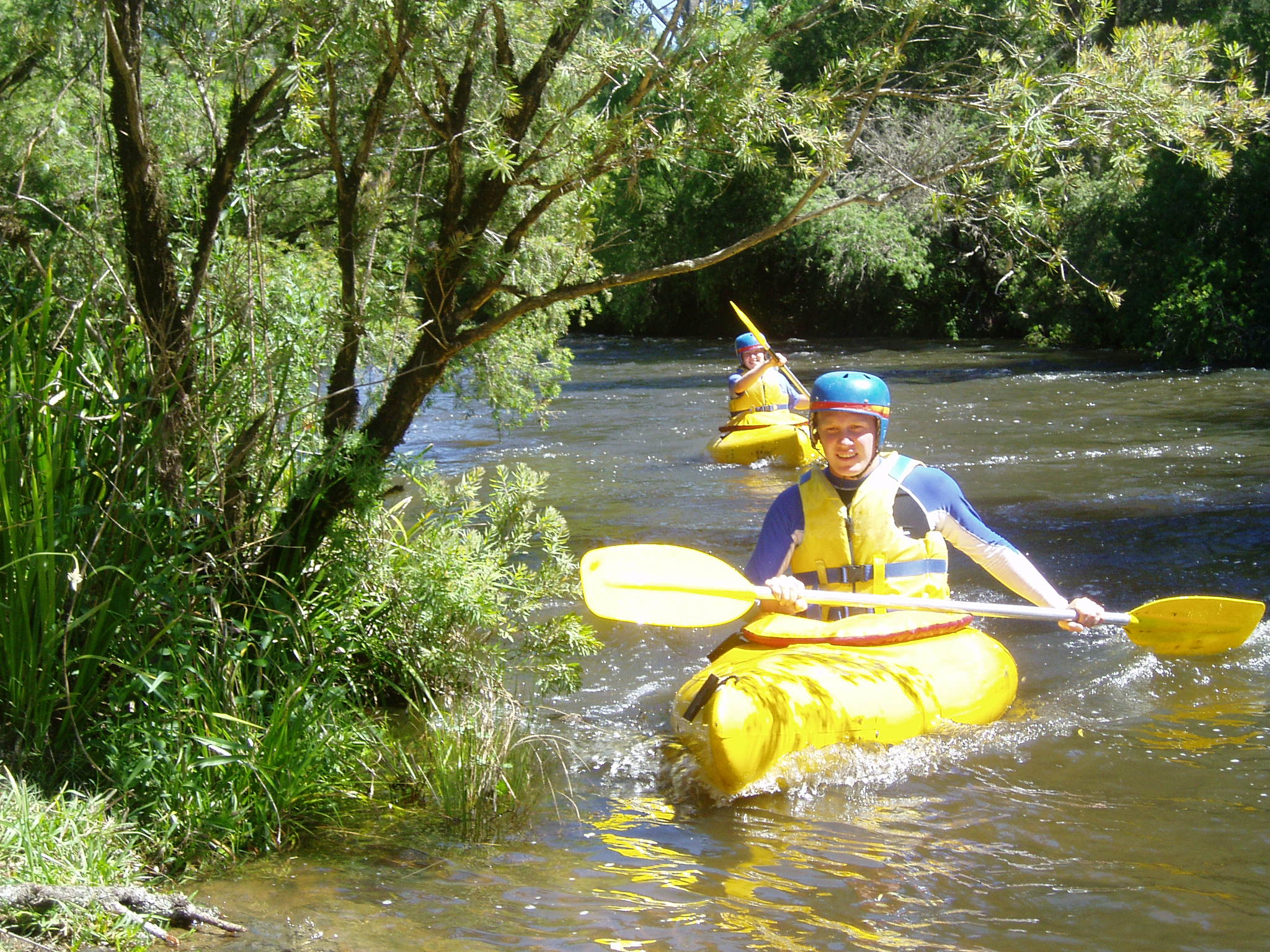×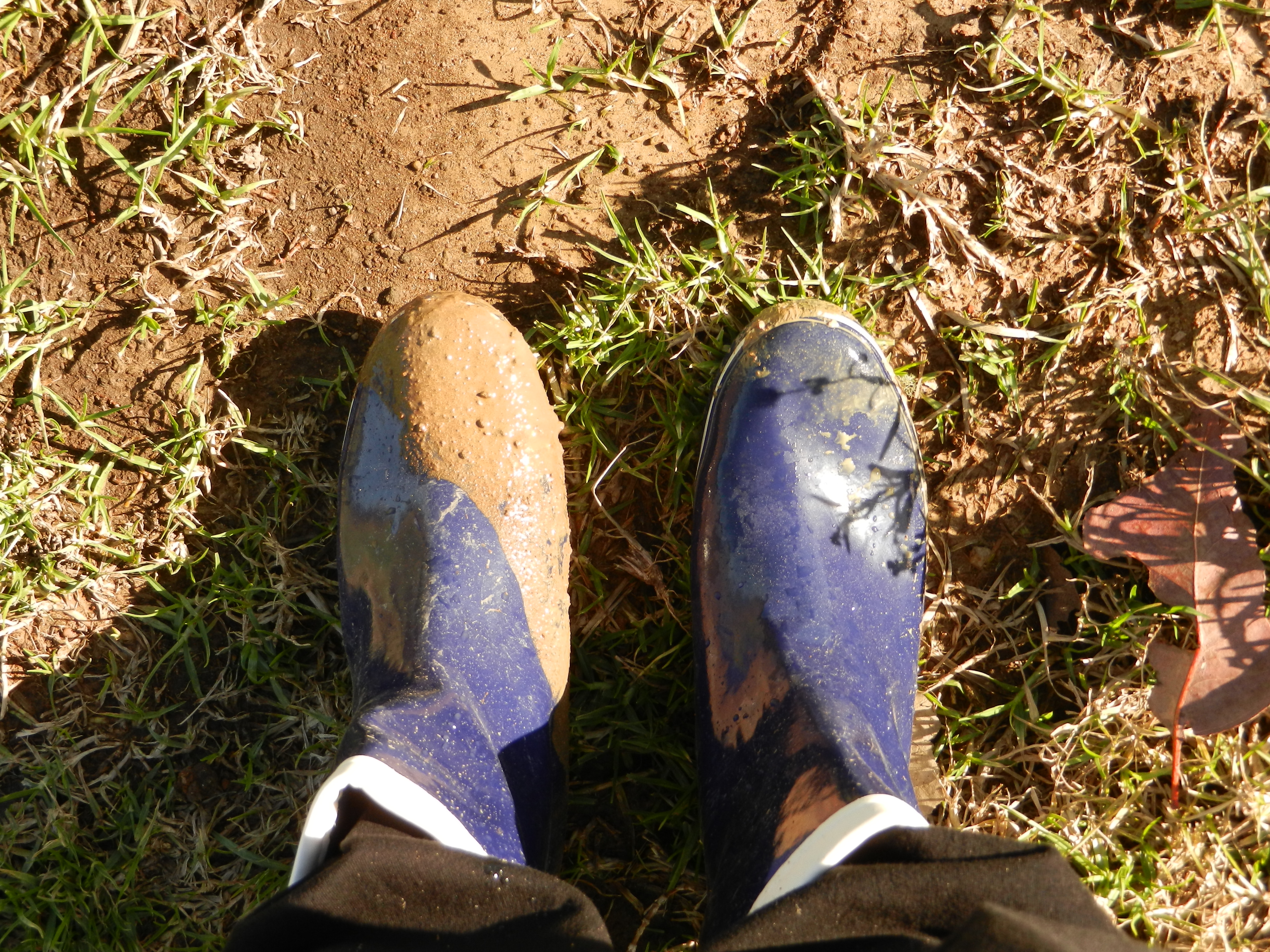×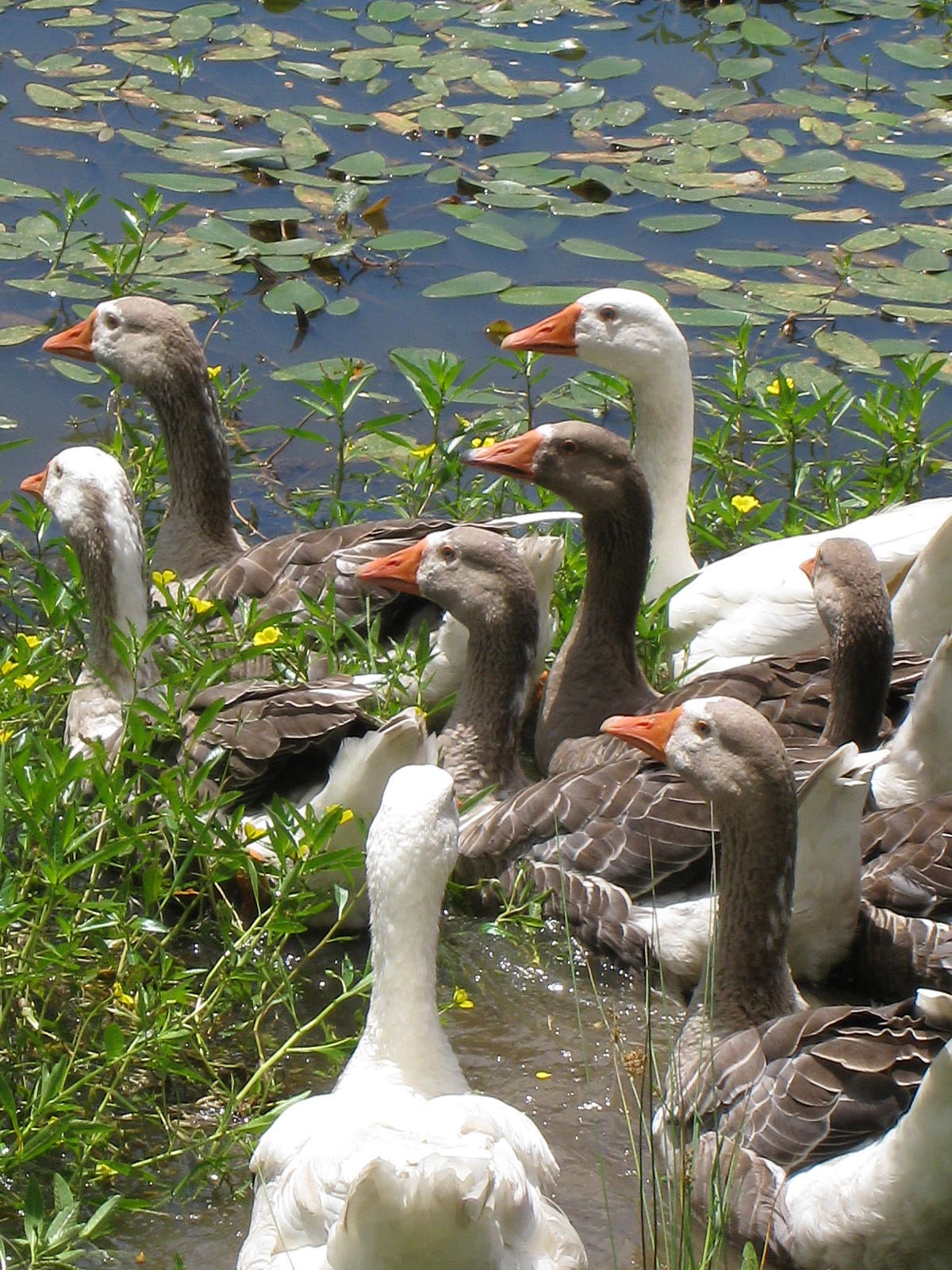××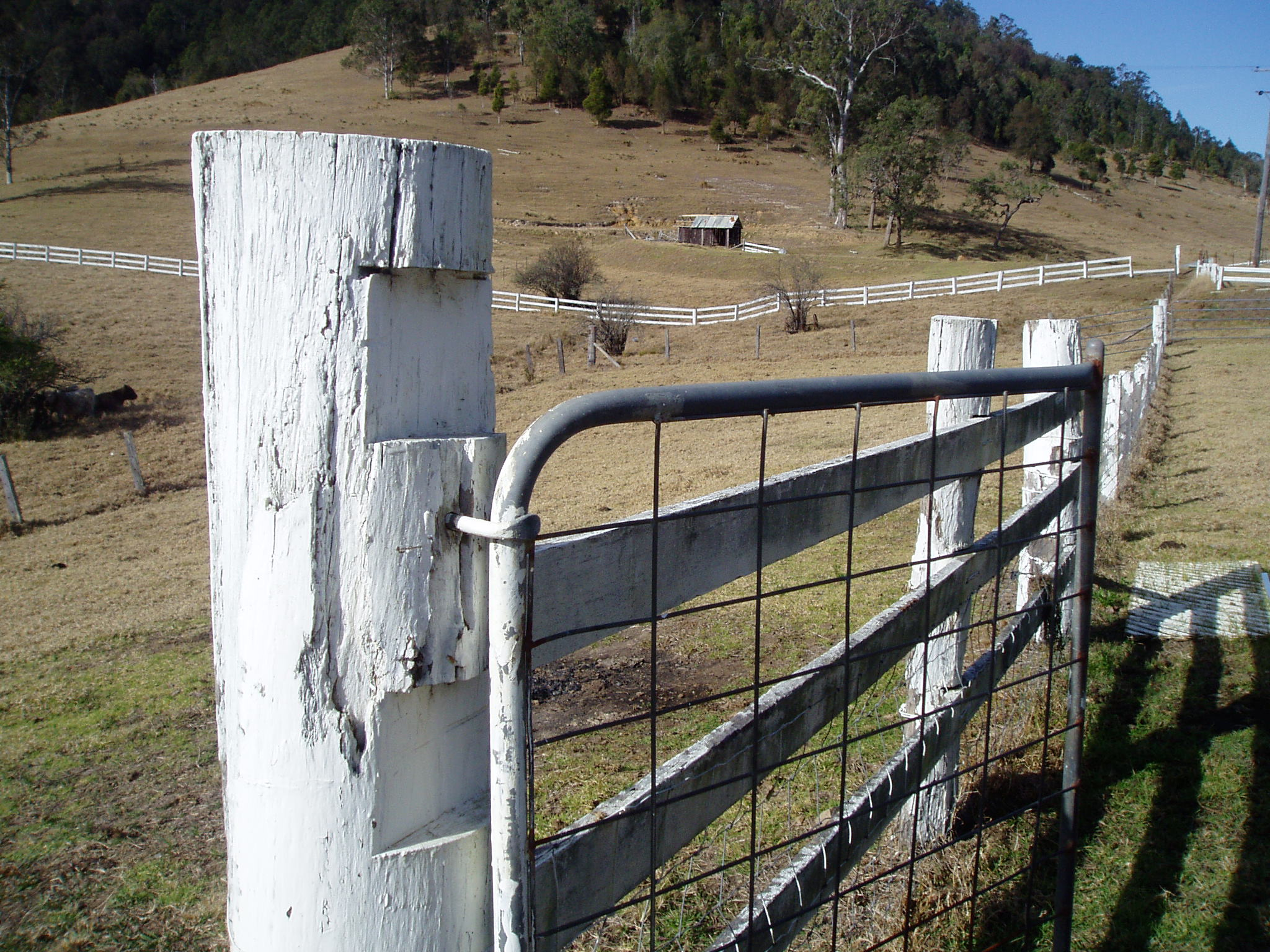×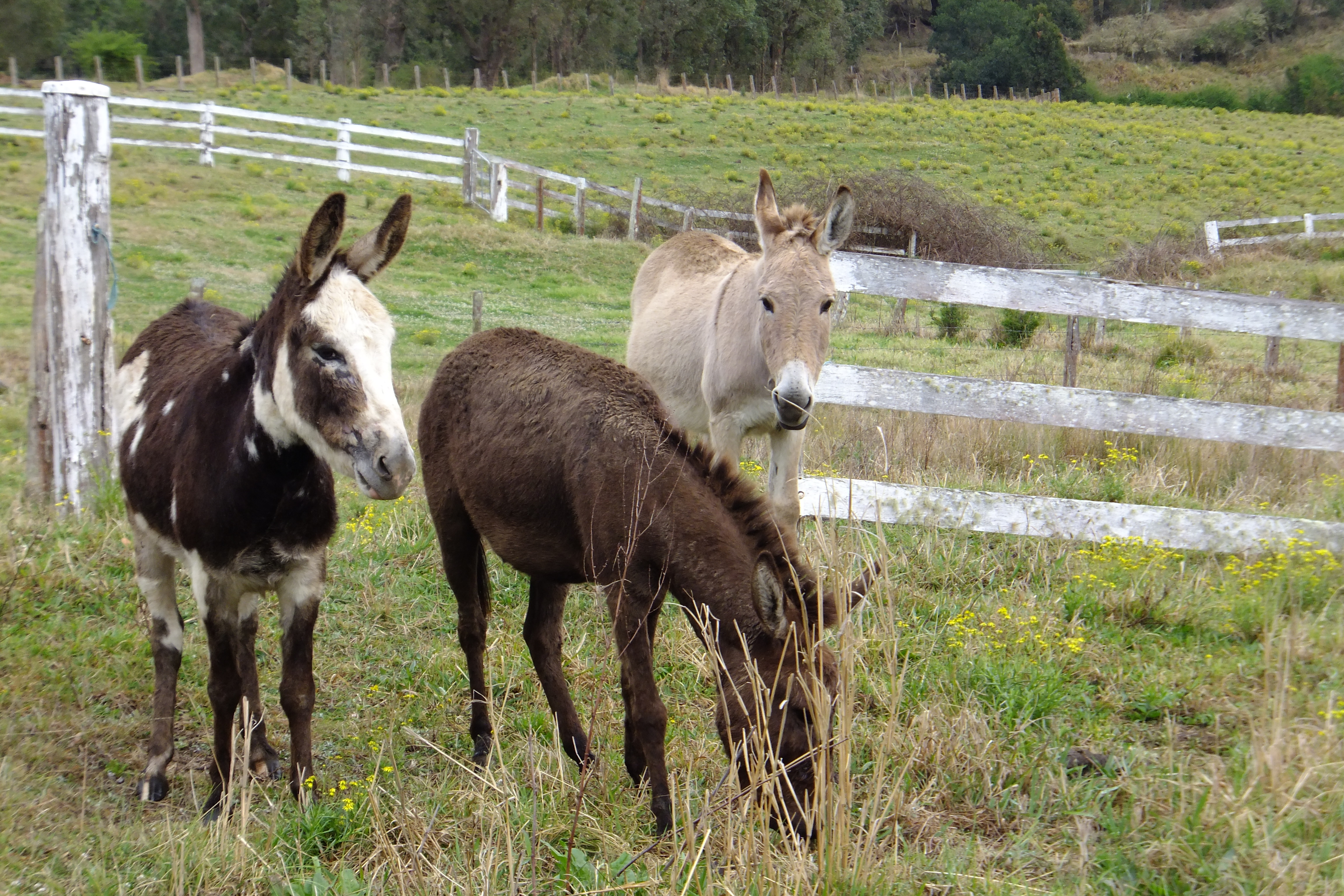×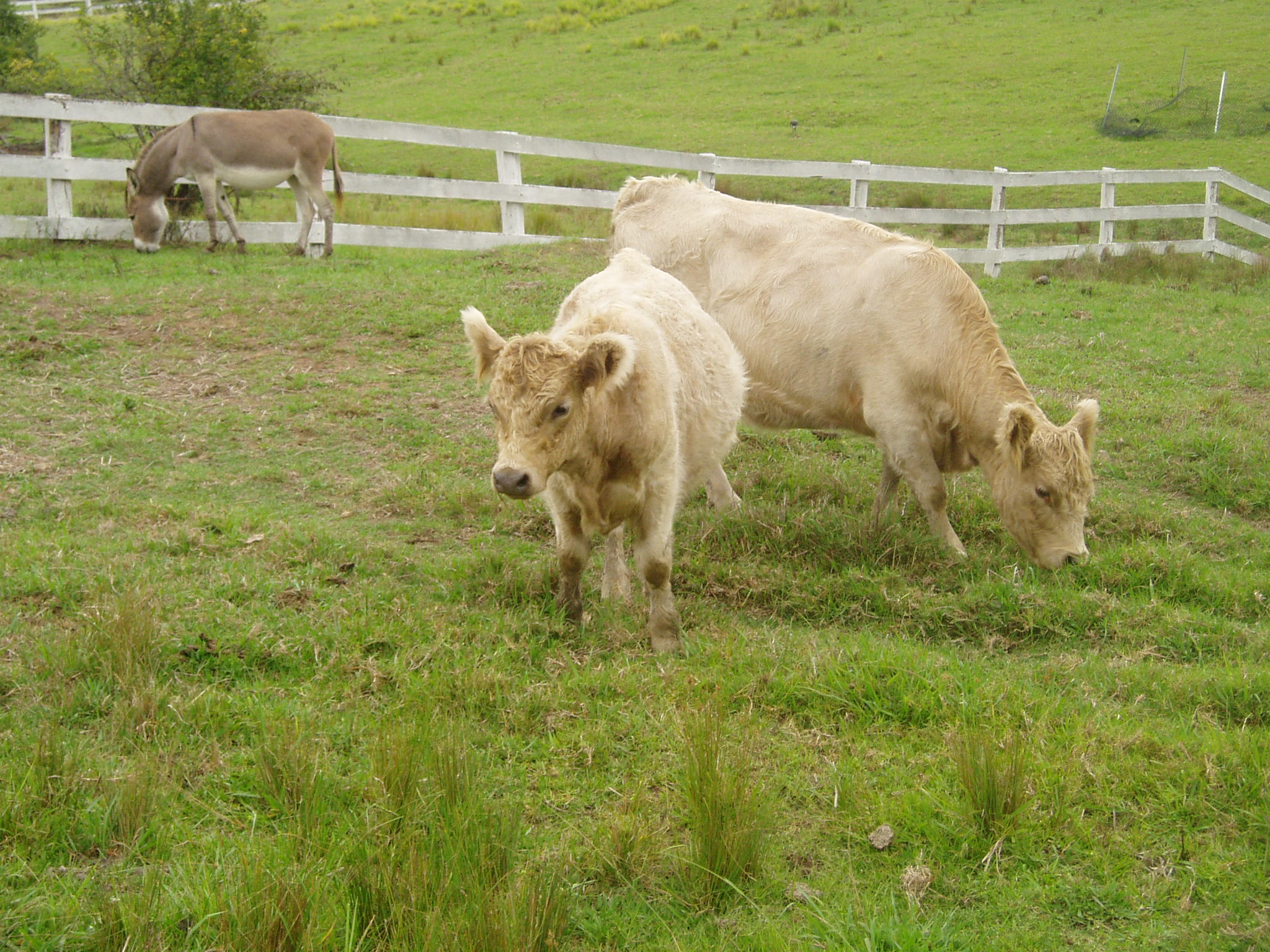××××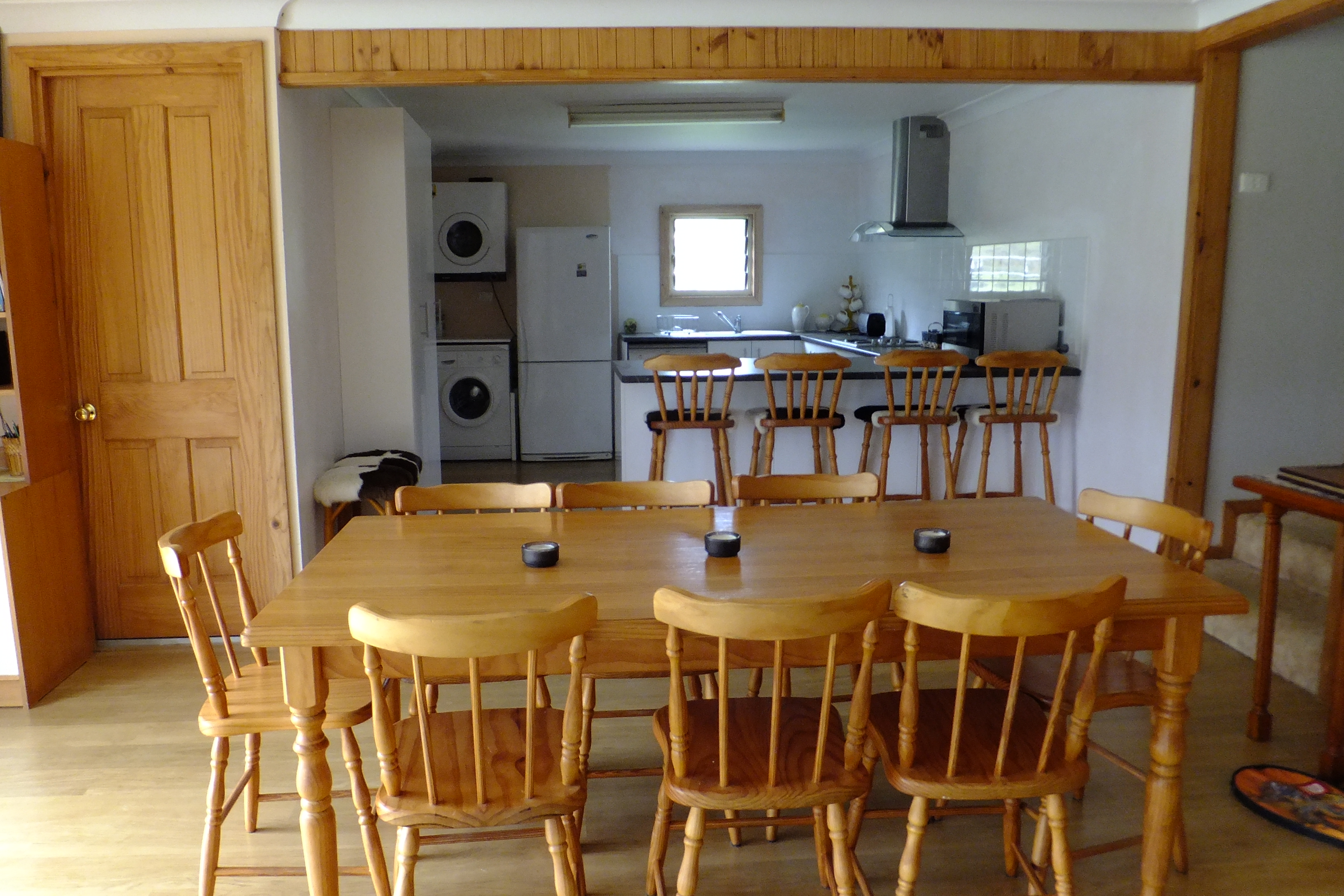××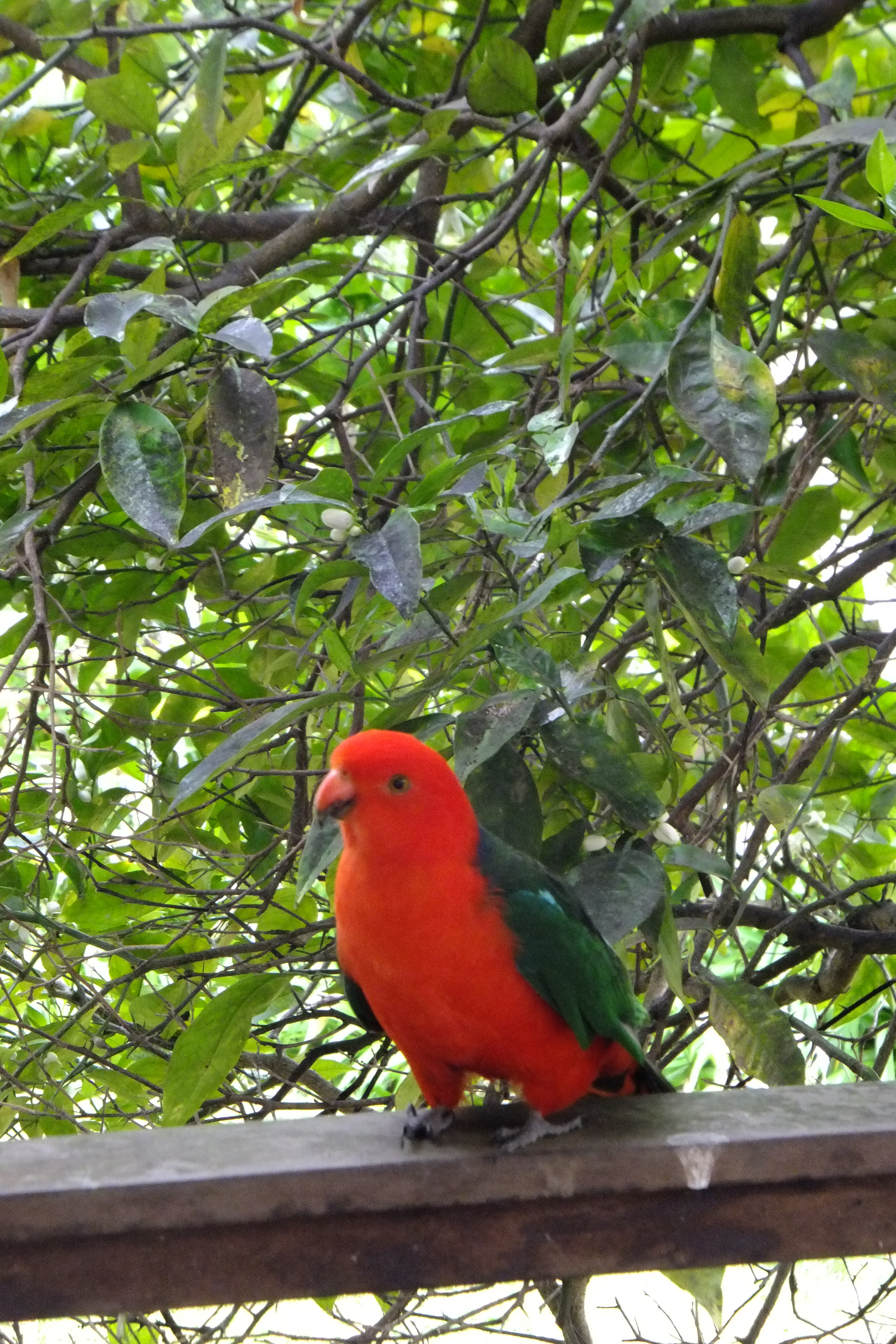×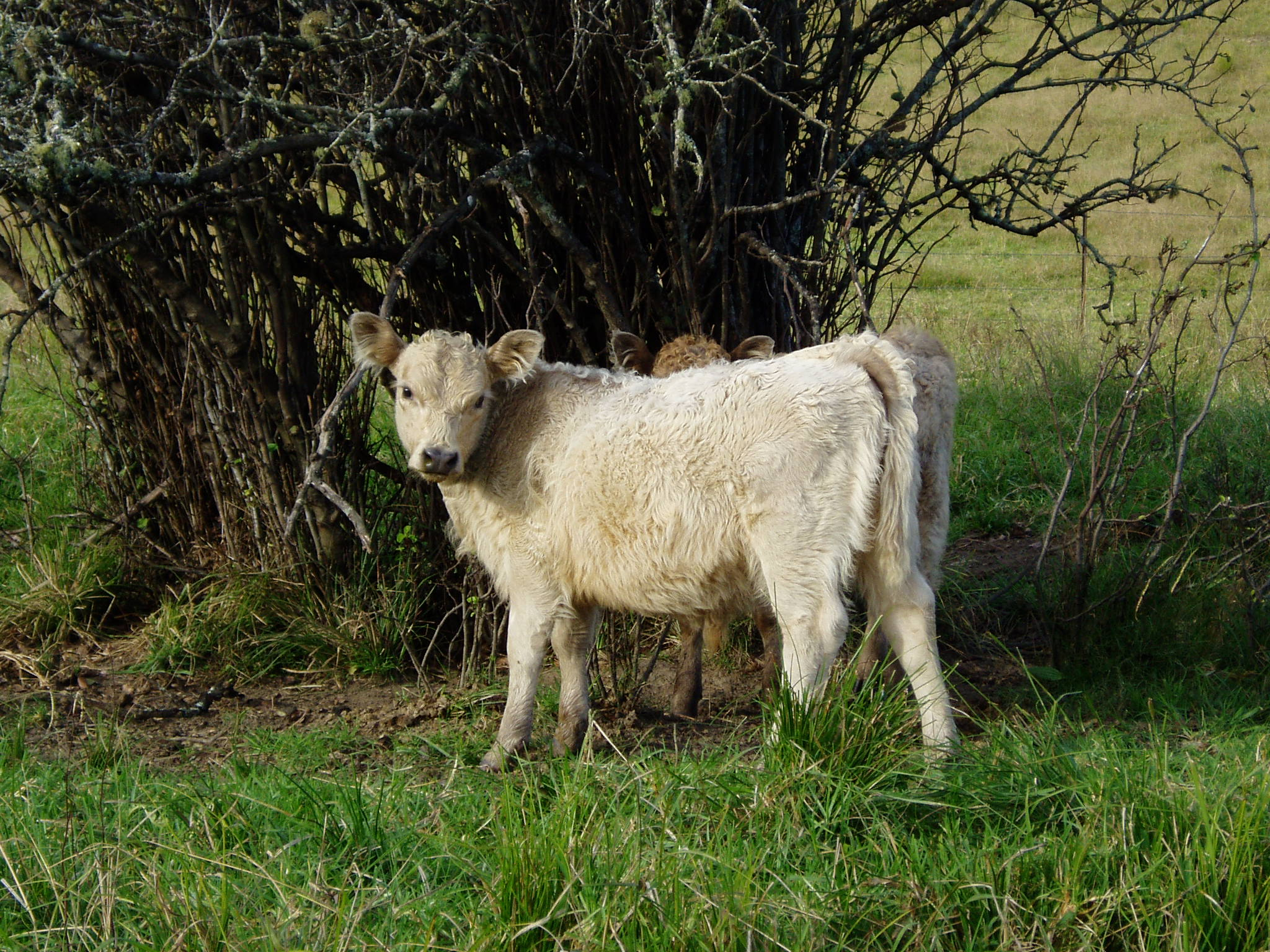×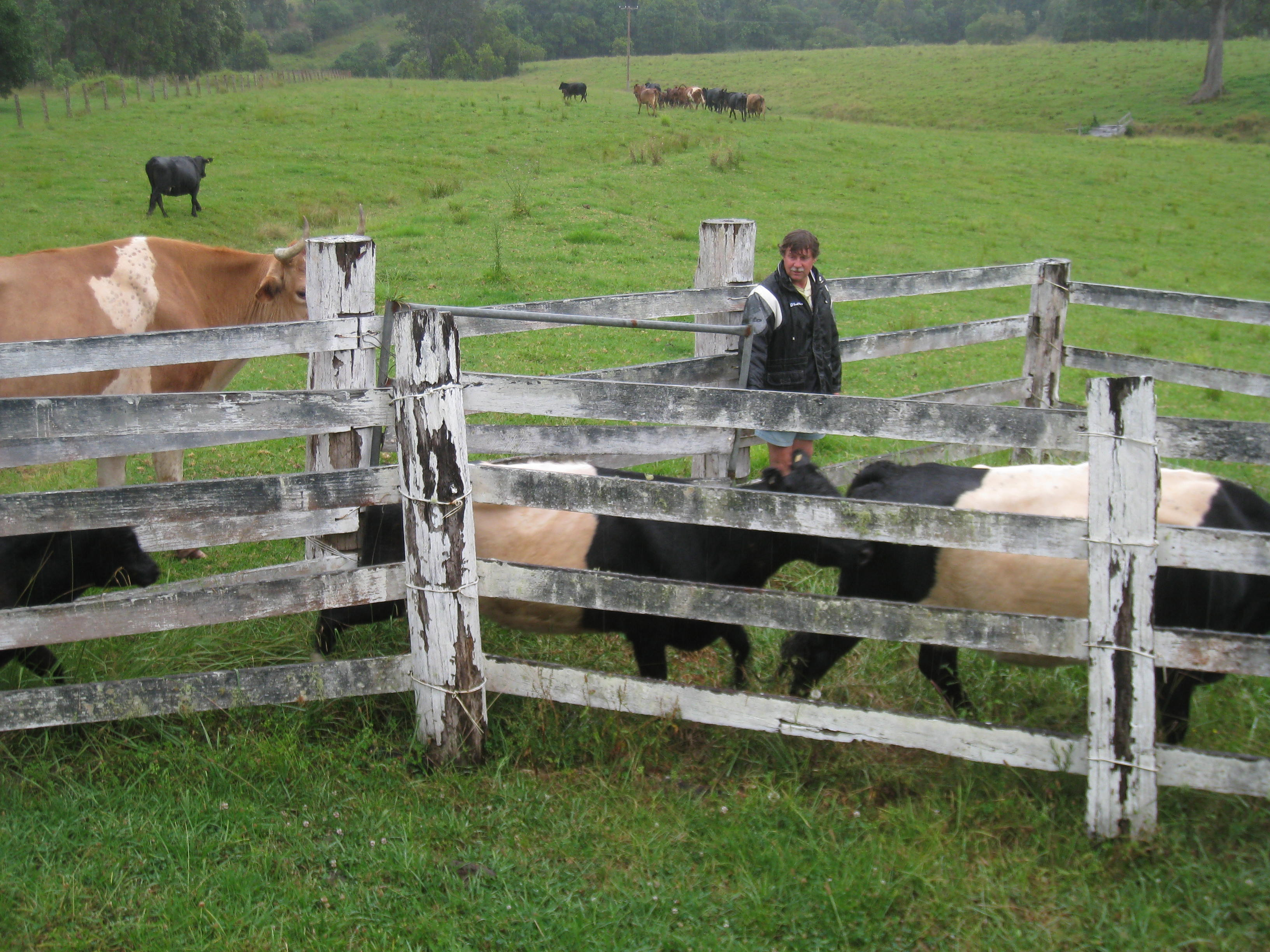×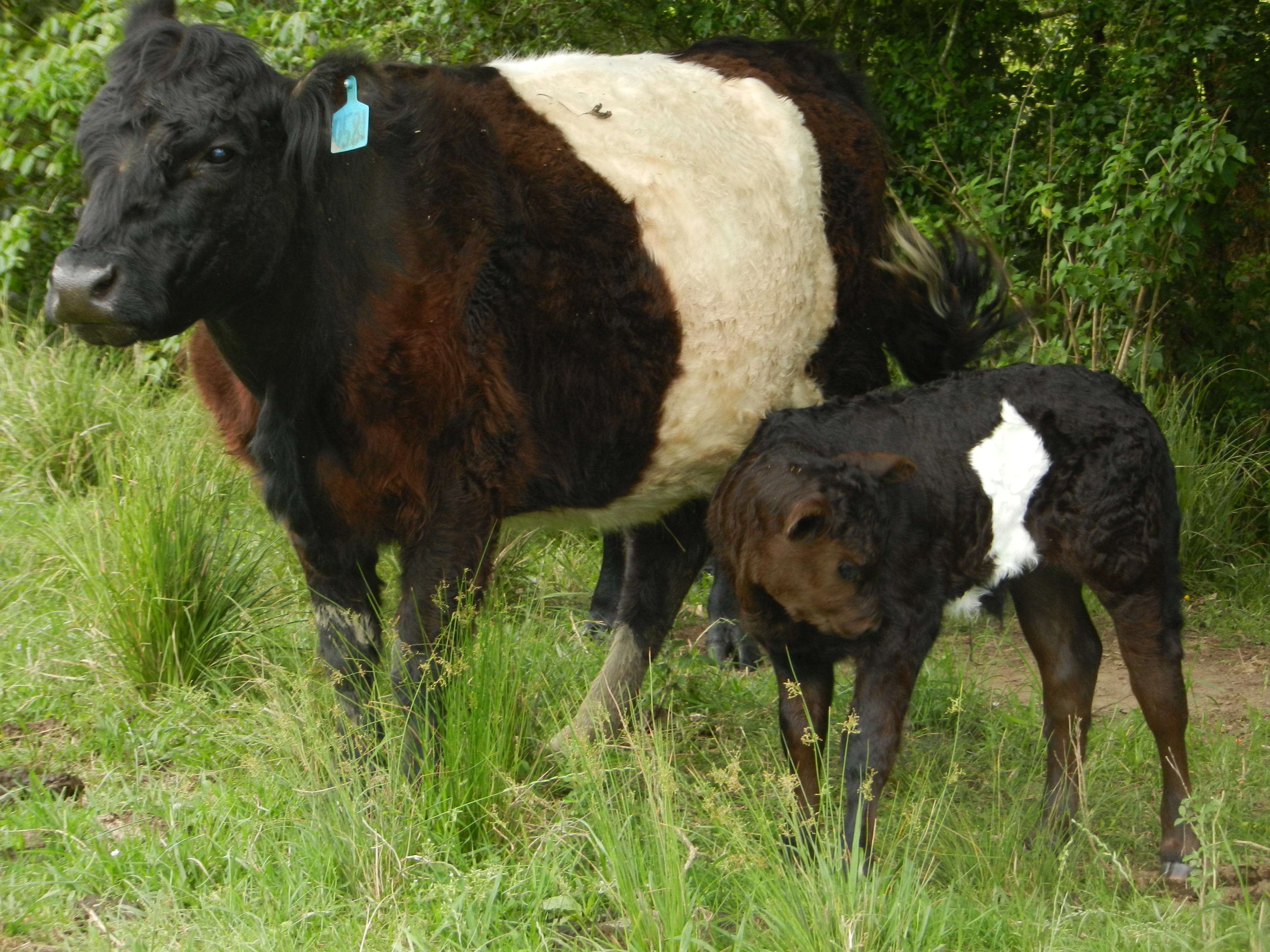××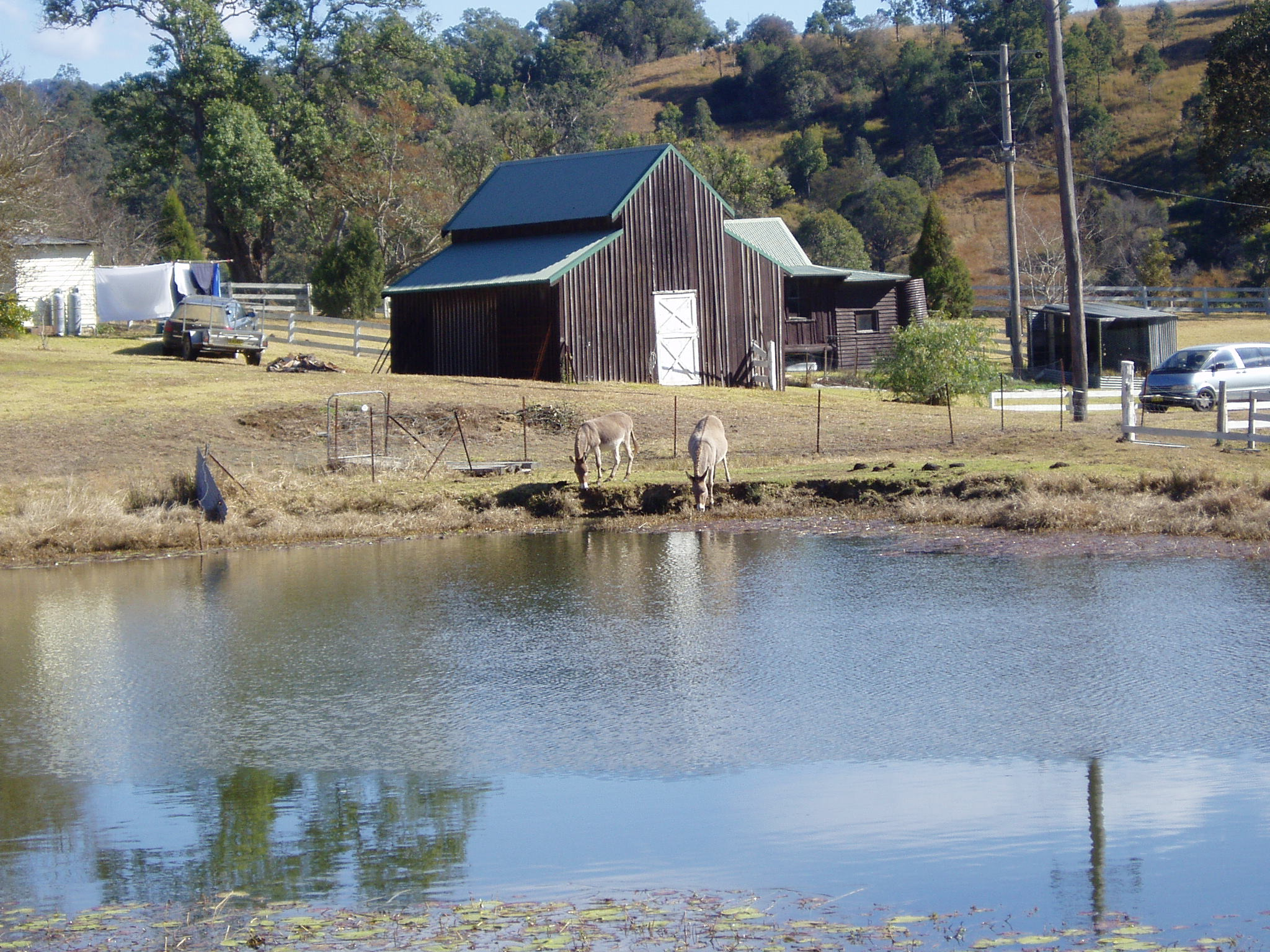×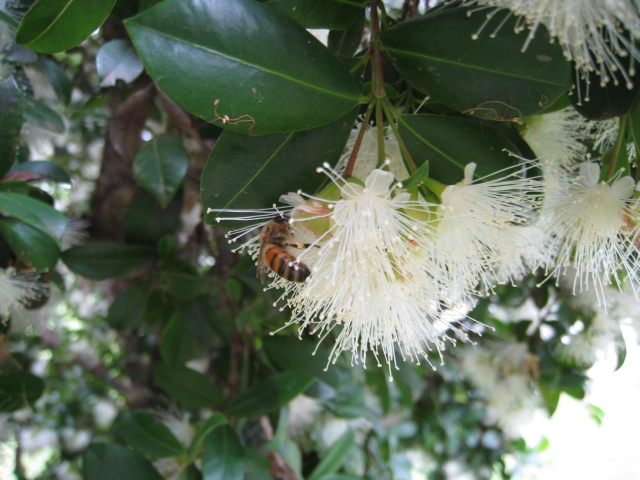×

## Book Now!

• Please enter a value between 2 and 12.
• Please enter a value less than or equal to 8.
×